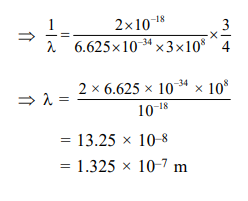Deepak Scored 45->99%ile with Bounce Back Crack Course. You can do it too!

# Based on the equation

Question:

Based on the equation

$\Delta \mathrm{E}=-2.0 \times 10^{-18} \mathrm{~J}\left(\frac{1}{\mathrm{n}_{2}^{2}}-\frac{1}{\mathrm{n}_{1}^{2}}\right)$

the wavelength of the light that must be absorbed to excite hydrogen electron from level $\mathrm{n}=$ 1 to level $\mathrm{n}=2$ will be $\left(\mathrm{h}=6.625 \times 10^{-34} \mathrm{Js}, \mathrm{C}=3 \times 10^{8} \mathrm{~ms}^{-1}\right)$

1. $2.650 \times 10^{-7} \mathrm{~m}$

2. $1.325 \times 10^{-7} \mathrm{~m}$

3. $1.325 \times 10^{-10} \mathrm{~m}$

4. $5.300 \times 10^{-10} \mathrm{~m}$

Correct Option: , 2

Solution:

$\frac{1}{\lambda}=\frac{2 \times 10^{-18}}{\mathrm{hc}}\left[\frac{1}{(1)^{2}}-\frac{1}{(2)^{2}}\right]$